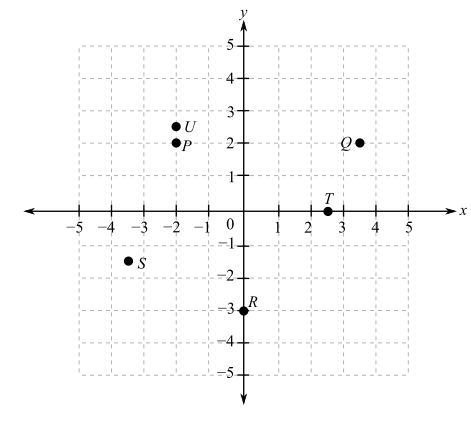Chapter 0.7, Problem 2E

Chapter
Section
Textbook Problem

Referring to the following figure, determine the coordinates of the indicated points as accurately as you can.To determine

The co-ordinates of the indicated points in the provided graph,Explanation

Given Information:

The provided graph is,

Consider the provided graph,

Start with the alphabetical order, the provided points are P, Q, R, S, T and U.

First take point P,

From the origin drop a vertical line of x=2 and horizontal line of y=2. Therefore, the coordinates are (2,2).

Consider next point Q,

Now from the origin, drop a vertical line of x=3.5 and horizontal line of y=2. Therefore, the coordinates are (3.5,2).

Consider next point R,

The point R has height of 3 units, so its coordinates are (0,3).

Similarly, for point S, T and U,

The coordinates are S(3

Still sussing out bartleby?

Check out a sample textbook solution.

See a sample solution

The Solution to Your Study Problems

Bartleby provides explanations to thousands of textbook problems written by our experts, many with advanced degrees!

Get Started

Find more solutions based on key concepts